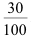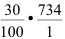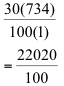SEARCH HOMEMath Central Quandaries & QueriesSubject: math Name: naheem Who are you: Student what is 40% of 936Hi Naheem,

When we find percents of numbers, we need to recall what a percentage is out of.  We know this is always out of 100.  As well we need to be able to translate the word 'of' which in fact just means multiplication.

Here is an example of solving a question similar to yours:
Find 30% of 734

Since we know percent is out of 100, we can change 30% to. As well we can replace the ‘of’ with multiplication and out question becomes:Now it’s just straight multiplying fractions which we can either cross cancel, or multiply straight across the tops and straight across the bottoms.  For this example, I'll do the latter.Now when we reduce, we end up with 220.2 and you’re done!

SaraMath Central is supported by the University of Regina and The Pacific Institute for the Mathematical Sciences.